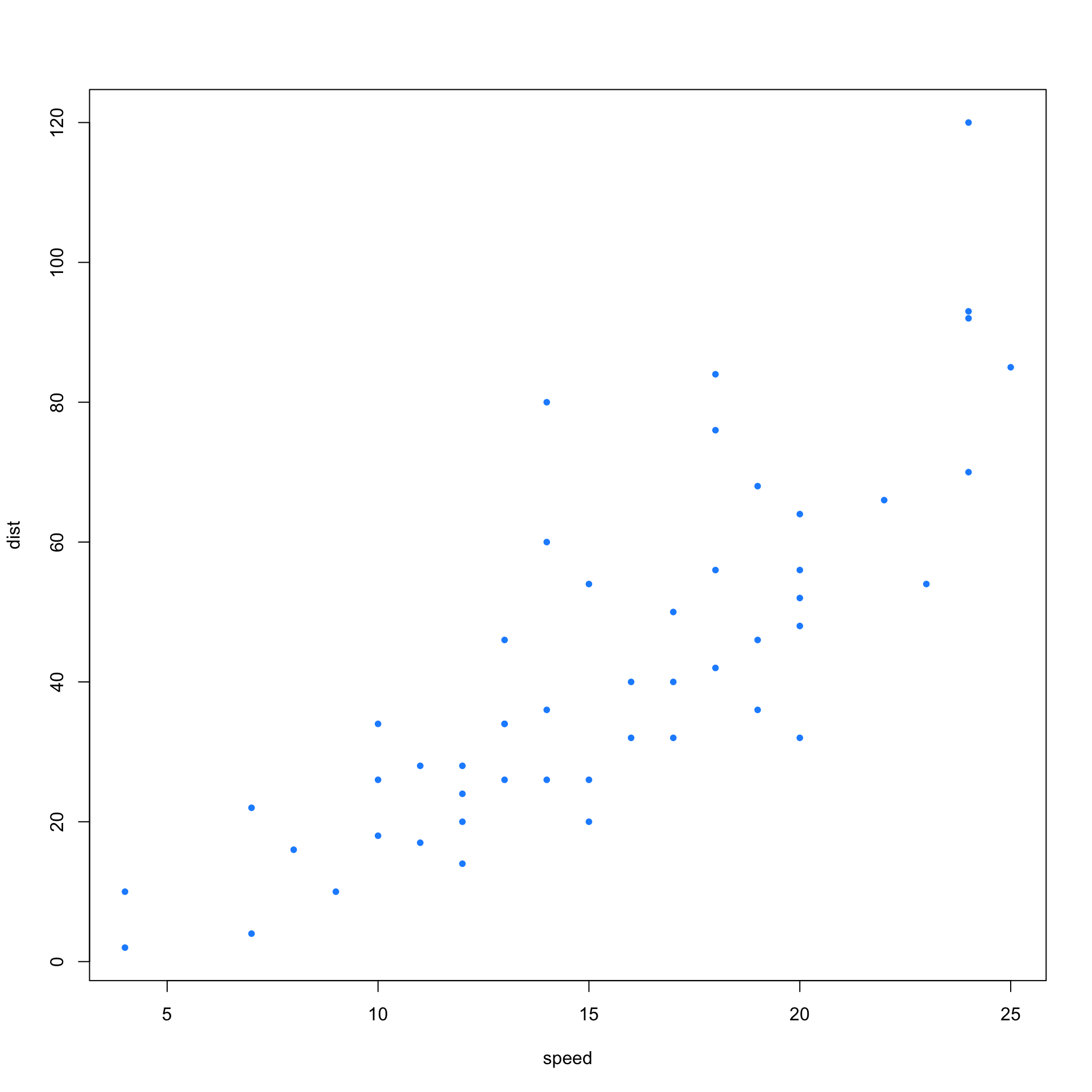# During Lecture

## Markdown

[Exercise] Write an unordered list of your classes this semester. Make the short course name (STAT 430) bold. Have the short course name link to the course description in the course explorer. Make the actual course title (Basics of Statistical Learning) italic.

• STAT 400 - Statistcis and Probability I
• STAT 432 - Basics of Statistical Learning

## Chunks

[Exercise] Add a chunk to this document that sums the integers from 1 to 100. It should display both the code and results after being knit. (Also, can you make this chunk only show output when knit? How about display the code, but don’t run the chunk when knit?)

sum(1:100)
##  5050

[Exercise] Add a chunk to this document that sums the integers from 1 to 100. It should display only the results after being knit.

##  5050

[Exercise] Read in lab02-data.csv as lab02_data.

lab02_data = read.csv("lab02-data.csv")

[Exercise] Report the first 10 rows of lab02_data as a table. Hint: check out the kable() function from the knitr packge.

y x
-0.4983332 -0.0649274
0.7906767 -0.2217219
-8.3984168 -1.7584576
0.1851392 -0.6899759
8.0737747 1.5137162
13.5180561 1.7224195
-3.6321427 -0.4312862
-6.2458735 -1.3646129
-2.6973023 -0.7202096
-5.8620015 -0.7721375

## Plotting

[Exercise] Create a scatterplot of dist vs speed for the cars data, a dataset built into R. Use chunk options to make the plot larger than the default.

plot(dist ~ speed, data = cars, col = "dodgerblue", pch = 20)# After Lecture

We probably went way too fast in lecture if this is your first time seeing rmarkdown. However, even if we went a lot slower, you would still need to engage a lot with rmarkdown to really get the hang of it. There are a number of things you should do to help get yourself up to speed:

• Go back through the in-class portion of this lab, finish anything that we did not finish in class.
• Check the solutions to this lab, even if you felt like you understood it the first time. Note that the solution file will contain a solution chunk option. This is not something you should use, this is just a by-product of the document creation method used.
• Watch the videos posted in Chapter 3 of R4SL, especially the playlist of rmarkdown.
• Read through the template document posted in Chapter 3 of R4SL. Try to change it a bit, then re-knit it.
• Do Homework 00!!!
• Try these additional exercises that will have there solutions posted as well.
• Post on Piazza if you have any questions.

## Randomization

rnorm(10)
set.seed(42)
rnorm(10)

[Exercise] Repeatedly run the (entire) two previous chunks. What’s the difference?

The first changes each time. The second is the same each time. This is because we have set a seed.

## LaTeX

With RMarkdown, you can not only mix R and markdown, but you can also use LaTeX. It can be used inline: $$y = x + 2$$ or in “equation mode,”

$a^2 + b^2 = c^2$

[Exercise] Write the density function for a normal distribution using LaTeX in equation mode.

• Hint: You can obtain the LaTeX for any equation in R4SL by right clicking it, selecting “Show Math As” then clicking “TeX Commands.”

$f(x | \mu, \sigma^2) = \frac{1}{\sigma\sqrt{2\pi}} \cdot \exp\left[\frac{-1}{2} \left(\frac{x - \mu}{\sigma}\right)^2 \right], \ \ \ -\infty < x < \infty, \ -\infty < \mu < \infty, \ \sigma > 0.$

## Chunk and Document Options

Some other things to try:

• Can you change the theme of this document?